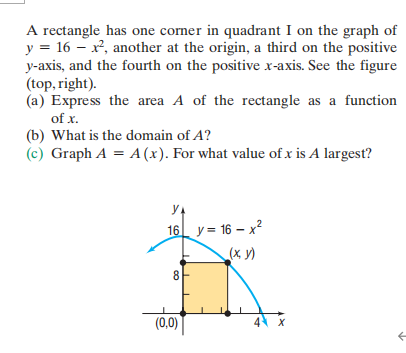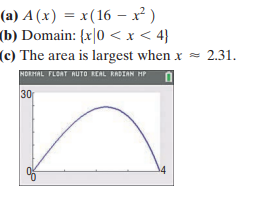### Still have math questions?

Algebra
QuestionA rectangle has one corner in quadrant I on the graph of

$$y = 16 - x ^ { 2 }$$ , another at the origin, a third on the positive

$$y$$ -axis, and the fourth on the positive $$x$$ -axis. See the figure (top, right). (a) Express the area $$A$$ of the rectangle as a function of $$x$$ . (b) What is the domain of $$A$$ ? (c) Graph $$A = A ( x )$$ . For what value of $$x$$ is $$A$$ largest?$$\left. \begin{array} { l } { \text { (a) } A ( x ) = x ( 16 - x ^ { 2 } ) } \\ { \text { (b) Domain: } \{ x | 0 < x < 4 \} } \\ { \text { (c) The area is largest when } x \approx 2.31 \text { . } } \end{array} \right.$$